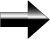Imaginary Numbers

Imaginary numbers are a special set of numbers that are different from the real numbers that we encounter in everyday life.  When a number has an imaginary part and a real part, we call the number a complex number.

We know that a negative number times a negative number gives us a positive number, and for that reason, we can not take the square root (or any even root, for that matter) of a negative number.  There is no real solution, but there is an imaginary solution.  Imaginary numbers are denoted by the lowercase letter “i”.  The definition of I is as follows:

i2 = -1

So, the square root of -1 is i.  We can now take negative square roots.

For example, √(-4) = √(4) * √(-1) = 2 * i = 2i.

Main Pre-Calculus Page